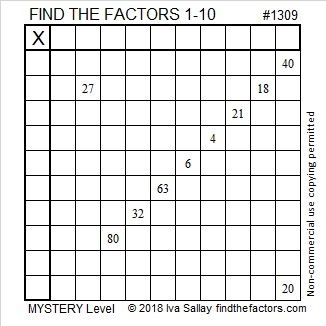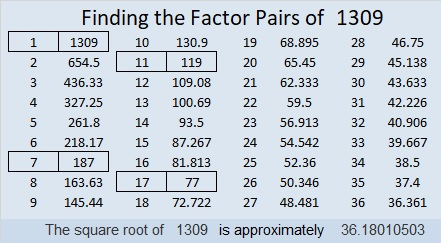# 1309 Mystery Level

This mystery level puzzle doesn’t look too hard, but is that deceptive? You will have to put your logical mind to work to know for sure.Print the puzzles or type the solution in this excel file: 10-factors-1302-1310

Now I’d like to share a few facts about 1309:

• 1309 is a composite number.
• Prime factorization: 1309 = 7 × 11 × 17
• The exponents in the prime factorization are 1, 1, and 1. Adding one to each and multiplying we get (1 + 1)(1 + 1)(1 + 1) = 2 × 2 × 2 = 8. Therefore 1309 has exactly 8 factors.
• Factors of 1309: 1, 7, 11, 17, 77, 119, 187, 1309
• Factor pairs: 1309 = 1 × 1309, 7 × 187, 11 × 119, or 17 × 77
• 1309 has no square factors that allow its square root to be simplified. √1309 ≈ 36.180111309 is the hypotenuse of a Pythagorean triple:
616-1155-1309 which is (8-15-17) times 77

As shown in their factor trees below, 1308, 1309, 1310, and 1311 each have three distinct prime numbers in their prime factorizations. They are the smallest set of four consecutive numbers with the same number of prime factors. 1309, 1310, and 1311 are also the smallest three consecutive numbers that have exactly the same number of factors and factor pairs. Thank you OEIS.org for alerting me to those facts.This site uses Akismet to reduce spam. Learn how your comment data is processed.